﻿ 基于事件的具有时变时滞多智能体系统的平均一致性

# 基于事件的具有时变时滞多智能体系统的平均一致性Event-Based Average Consensus of Multi-Agent Systems with Time-Varying Delays

Abstract: In this paper, the method of periodic sampling and event control is used to analyze the delay ro-bustness of the event-triggered average consensus problem for first-order multi-agent systems with time-varying communication delays. By designing consensus protocol and event-triggering conditions, all agents can achieve consensus under lower communication frequency. By using Lyapunov stability theory, it is proved that average consensus of multi-agent systems can be achieved and the obtained results improve the existing ones. Finally, the validity of the theoretical results is illustrated by numerical simulations.

1. 引言

2. 问题陈述

2.1. 图论知识

$\stackrel{^}{A}=\left[{\stackrel{^}{a}}_{ij}\right]\in {R}^{n×n}$${\stackrel{^}{a}}_{ij}={\stackrel{^}{a}}_{ji}=\left({a}_{ij}+{a}_{ji}\right)/2$$\stackrel{^}{G}$ 的拉普拉斯矩阵记为 $\stackrel{^}{L}$

2.2. 模型建立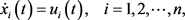(1)

${u}_{i}\left(t\right)=\left\{\begin{array}{l}0,\text{\hspace{0.17em}}\text{\hspace{0.17em}}t\in \left[0,\tau \left(0\right)\right)，\hfill \\ -\underset{j\in {N}_{i}}{\sum }{a}_{ij}\left[{\stackrel{^}{x}}_{i}\left(t-\stackrel{^}{\tau }\left(t\right)\right)-{\stackrel{^}{x}}_{j}\left(t-\stackrel{^}{\tau }\left(t\right)\right)\right],\text{\hspace{0.17em}}\text{\hspace{0.17em}}t\in \left[\tau \left(0\right),+\infty \right).\hfill \end{array}$ (2)

$\stackrel{˙}{x}\left(t\right)=-L\stackrel{^}{x}\left(t-\stackrel{^}{\tau }\left(t\right)\right),$ (3)

${e}_{i}^{2}\left({t}_{k}^{i}+lh\right)>\left({\sigma }^{2}/\underset{j\in {N}_{i}}{\sum }{a}_{ij}\right){\stackrel{^}{y}}_{i}^{2}\left({t}_{k}^{i}+\left(l-1\right)h\right),l\in {Z}^{+},$ (4)

${\stackrel{^}{x}}_{i}\left({t}_{k+1}^{i}\right)={x}_{i}\left({t}_{k+1}^{i}\right)$

3. 主要结果

${x}^{\text{T}}Lx\ge {\alpha }_{2}{‖x-\frac{1}{n}\underset{i=1}{\overset{n}{\sum }}{x}_{i}{1}_{n}‖}^{2},$

${x}^{\text{T}}\left(kh\right){L}^{\text{T}}Lx\left(kh\right)\le \frac{{\beta }_{n}}{{\alpha }_{2}}{x}^{\text{T}}\left(kh\right)Lx\left(kh\right),$

$\begin{array}{l}-\left[\left(\frac{{\beta }_{n}}{2{\alpha }_{2}}\tau \left(\left(k+1\right)h\right)+\sigma \right)\left(h+\tau \left(\left(k+2\right)h\right)-\tau \left(\left(k+1\right)h\right)\right)\\ +\left(h+\tau \left(\left(k+1\right)h\right)-\tau \left(kh\right)\right)\left(\frac{{\beta }_{n}}{2{\alpha }_{2}}\left(h+\tau \left(\left(k+1\right)h\right)\right)-1+\sigma \right)\right]\ge {\epsilon }_{0},\end{array}$ (5)

$V\left(t\right)=V\left(x\left(t\right)\right)=\frac{1}{2}{x}^{\text{T}}\left(t\right)x\left(t\right),t\in \left[\tau \left(0\right),+\infty \right).$

$\begin{array}{c}x\left(t\right)=x\left(kh+\tau \left(kh\right)\right)-\left(t-\left(kh+\tau \left(kh\right)\right)\right)L\stackrel{^}{x}\left(kh\right)\\ =x\left(kh\right)-\tau \left(kh\right)L\stackrel{^}{x}\left(\left(k-1\right)h\right)-\left(t-\left(kh+\tau \left(kh\right)\right)\right)L\stackrel{^}{x}\left(kh\right),k\in {Z}^{+}.\end{array}$ (6)

$t\in \left[kh+\tau \left(kh\right),\left(k+1\right)h+\tau \left(\left(k+1\right)h\right)\right)$，对 $V\left(t\right)$ 进行求导，结合(6)可以得到(7)

$\begin{array}{c}|{\stackrel{^}{x}}^{\text{T}}\left(\left(k-1\right)h\right){L}^{\text{T}}L\stackrel{^}{x}\left(kh\right)|\le \frac{1}{2}\left({\stackrel{^}{x}}^{\text{T}}\left(\left(k-1\right)h\right){L}^{\text{T}}L\stackrel{^}{x}\left(\left(k-1\right)h\right)+{\stackrel{^}{x}}^{\text{T}}\left(kh\right){L}^{\text{T}}L\stackrel{^}{x}\left(kh\right)\right)\\ \le \frac{{\beta }_{n}}{2{\alpha }_{2}}\left({\stackrel{^}{x}}^{\text{T}}\left(\left(k-1\right)h\right)L\stackrel{^}{x}\left(\left(k-1\right)h\right)+{\stackrel{^}{x}}^{\text{T}}\left(kh\right)L\stackrel{^}{x}\left(kh\right)\right),k\in {Z}^{+}.\end{array}$ (8)

$\begin{array}{c}|{e}^{\text{T}}\left(kh\right)L\stackrel{^}{x}\left(kh\right)|=|\underset{i=1}{\overset{n}{\sum }}\left[{e}_{i}\left(kh\right)\underset{j\in {N}_{i}}{\sum }{a}_{ij}\left({\stackrel{^}{x}}_{i}\left(kh\right)-{\stackrel{^}{x}}_{j}\left(kh\right)\right)\right]|\\ \le \frac{1}{2}\underset{i=1}{\overset{n}{\sum }}\underset{j\in {N}_{i}}{\sum }\left[\frac{{e}_{i}^{2}\left(kh\right){a}_{ij}}{\sigma }+\sigma {a}_{ij}{\left({\stackrel{^}{x}}_{i}\left(kh\right)-{\stackrel{^}{x}}_{j}\left(kh\right)\right)}^{2}\right]\text{ }\text{ }.\end{array}$ (9)

$\begin{array}{l}V\left(\left(k+1\right)h+\tau \left(\left(k+1\right)h\right)\right)\\ =V\left(kh+\tau \left(kh\right)\right)-\left(h+\tau \left(\left(k+1\right)h\right)-\tau \left(kh\right)\right){\stackrel{^}{x}}^{\text{T}}\left(kh\right)L\stackrel{^}{x}\left(kh\right)\\ \text{\hspace{0.17em}}\text{\hspace{0.17em}}-\left(h+\tau \left(\left(k+1\right)h\right)-\tau \left(kh\right)\right){e}^{\text{T}}\left(kh\right)L\stackrel{^}{x}\left(kh\right)\\ \text{\hspace{0.17em}}\text{\hspace{0.17em}}+\tau \left(kh\right)\left(h+\tau \left(\left(k+1\right)h\right)-\tau \left(kh\right)\right){\stackrel{^}{x}}^{\text{T}}\left(\left(k-1\right)h\right){L}^{\text{T}}L\stackrel{^}{x}\left(kh\right)\\ \text{\hspace{0.17em}}\text{\hspace{0.17em}}+\frac{1}{2}{\left(h+\tau \left(\left(k+1\right)h\right)-\tau \left(kh\right)\right)}^{2}{\stackrel{^}{x}}^{\text{T}}\left(kh\right){L}^{\text{T}}L\stackrel{^}{x}\left( k h \right)\end{array}$

$\begin{array}{l}\le V\left(kh+\tau \left(kh\right)\right)-\left(h+\tau \left(\left(k+1\right)h\right)-\tau \left(kh\right)\right){\stackrel{^}{x}}^{\text{T}}\left(kh\right)L\stackrel{^}{x}\left(kh\right)\\ \text{\hspace{0.17em}}\text{\hspace{0.17em}}+\frac{1}{2}\left(h+\tau \left(\left(k+1\right)h\right)-\tau \left(kh\right)\right)\underset{i=1}{\overset{n}{\sum }}\underset{j\in {N}_{i}}{\sum }\left[\frac{{e}_{i}^{2}\left(kh\right){a}_{ij}}{\sigma }+\sigma {a}_{ij}{\left({\stackrel{^}{x}}_{i}\left(kh\right)-{\stackrel{^}{x}}_{j}\left(kh\right)\right)}^{2}\right]\\ \text{\hspace{0.17em}}\text{\hspace{0.17em}}+\frac{{\beta }_{n}}{2{\alpha }_{2}}\tau \left(kh\right)\left(h+\tau \left(\left(k+1\right)h\right)-\tau \left(kh\right)\right){\stackrel{^}{x}}^{\text{T}}\left(\left(k-1\right)h\right)L\stackrel{^}{x}\left(\left(k-1\right)h\right)\\ \text{\hspace{0.17em}}\text{\hspace{0.17em}}+\frac{{\beta }_{n}}{2{\alpha }_{2}}\tau \left(kh\right)\left(h+\tau \left(\left(k+1\right)h\right)-\tau \left(kh\right)\right){\stackrel{^}{x}}^{\text{T}}\left(kh\right)L\stackrel{^}{x}\left(kh\right)\\ \text{\hspace{0.17em}}\text{\hspace{0.17em}}+\frac{{\beta }_{n}}{2{\alpha }_{2}}{\left(h+\tau \left(\left(k+1\right)h\right)-\tau \left(kh\right)\right)}^{2}{\stackrel{^}{x}}^{\text{T}}\left(kh\right)L\stackrel{^}{x}\left( k h \right)\end{array}$

$\begin{array}{l}=V\left(kh+\tau \left(kh\right)\right)+\frac{1}{2}\left(h+\tau \left(\left(k+1\right)h\right)-\tau \left(kh\right)\right)\underset{i=1}{\overset{n}{\sum }}\underset{j\in {N}_{i}}{\sum }\left[\frac{{e}_{i}^{2}\left(kh\right){a}_{ij}}{\sigma }+\sigma {a}_{ij}{\left({\stackrel{^}{x}}_{i}\left(kh\right)-{\stackrel{^}{x}}_{j}\left(kh\right)\right)}^{2}\right]\\ \text{\hspace{0.17em}}\text{\hspace{0.17em}}\text{+}\frac{{\beta }_{n}}{2{\alpha }_{2}}\tau \left(kh\right)\left(h+\tau \left(\left(k+1\right)h\right)-\tau \left(kh\right)\right){\stackrel{^}{x}}^{\text{T}}\left(\left(k-1\right)h\right)L\stackrel{^}{x}\left(\left(k-1\right)h\right)\\ \text{\hspace{0.17em}}\text{\hspace{0.17em}}+\left(h+\tau \left(\left(k+1\right)h\right)-\tau \left(kh\right)\right)\left(\frac{{\beta }_{n}}{2{\alpha }_{2}}\left(h+\tau \left(\left(k+1\right)h\right)\right)-1\right){\stackrel{^}{x}}^{\text{T}}\left(kh\right)L\stackrel{^}{x}\left(kh\right),k\in {Z}^{+}.\end{array}$

$\begin{array}{l}V\left(\left(k+1\right)h+\tau \left(\left(k+1\right)h\right)\right)\\ =V\left(\tau \left(0\right)\right)+{\int }_{\tau \left(0\right)}^{h+\tau \left(h\right)}\stackrel{˙}{V}\left(t\right)\text{d}t+{\int }_{h+\tau \left(h\right)}^{2h+\tau \left(2h\right)}\stackrel{˙}{V}\left(t\right)\text{d}t+\cdots +{\int }_{kh+\tau \left(kh\right)}^{\left(k+1\right)h+\tau \left(\left(k+1\right)h\right)}\stackrel{˙}{V}\left(t\right)\text{d}t,\end{array}$

$\begin{array}{l}V\left(\left(k+1\right)h+\tau \left(\left(k+1\right)h\right)\right)\\ \le V\left(\tau \left(0\right)\right)+{\int }_{\tau \left(0\right)}^{h+\tau \left(h\right)}\stackrel{˙}{V}\left(t\right)\text{d}t\\ \text{\hspace{0.17em}}\text{\hspace{0.17em}}+\underset{q=1}{\overset{k}{\sum }}\frac{1}{2}\left(h+\tau \left(\left(q+1\right)h\right)-\tau \left(qh\right)\right)\underset{i=1}{\overset{n}{\sum }}\underset{j\in {N}_{i}}{\sum }\left[\frac{{e}_{i}^{2}\left(qh\right){a}_{ij}}{\sigma }+\sigma {a}_{ij}{\left({\stackrel{^}{x}}_{i}\left(qh\right)-{\stackrel{^}{x}}_{j}\left(qh\right)\right)}^{2}\right]\\ \text{\hspace{0.17em}}\text{\hspace{0.17em}}+\frac{{\beta }_{n}}{2{\alpha }_{2}}\underset{q=1}{\overset{k}{\sum }}\tau \left(qh\right)\left(h+\tau \left(\left(q+1\right)h\right)-\tau \left(qh\right)\right){\stackrel{^}{x}}^{\text{T}}\left(\left(q-1\right)h\right)L\stackrel{^}{x}\left(\left(q-1\right)h\right)\\ \text{\hspace{0.17em}}\text{\hspace{0.17em}}+\underset{q=1}{\overset{k}{\sum }}\left(h+\tau \left(\left(q+1\right)h\right)-\tau \left(qh\right)\right)\left[\frac{{\beta }_{n}}{2{\alpha }_{2}}\left(h+\tau \left(\left(k+1\right)h\right)\right)-1\right]{\stackrel{^}{x}}^{\text{T}}\left(kh\right)L\stackrel{^}{x}\left( k h \right)\end{array}$

$\begin{array}{l}=V\left(\tau \left(0\right)\right)+{\int }_{\tau \left(0\right)}^{h+\tau \left(h\right)}\stackrel{˙}{V}\left(t\right)\text{d}t+\frac{{\beta }_{n}}{2{\alpha }_{2}}\tau \left(h\right)\left(h+\tau \left(2h\right)-\tau \left(h\right)\right){\stackrel{^}{x}}^{\text{T}}\left(0\right)L\stackrel{^}{x}\left(0\right)\\ \text{\hspace{0.17em}}\text{\hspace{0.17em}}+\underset{q=1}{\overset{k}{\sum }}\frac{1}{2}\left(h+\tau \left(\left(q+1\right)h\right)-\tau \left(qh\right)\right)\underset{i=1}{\overset{n}{\sum }}\underset{j\in {N}_{i}}{\sum }\left[\frac{{e}_{i}^{2}\left(qh\right){a}_{ij}}{\sigma }+\sigma {a}_{ij}{\left({\stackrel{^}{x}}_{i}\left(qh\right)-{\stackrel{^}{x}}_{j}\left(qh\right)\right)}^{2}\right]\\ \text{\hspace{0.17em}}\text{\hspace{0.17em}}+\left(h+\tau \left(\left(k+1\right)h\right)-\tau \left(kh\right)\right)\left[\frac{{\beta }_{n}}{2{\alpha }_{2}}\left(h+\tau \left(\left(k+1\right)h\right)\right)-1\right]{\stackrel{^}{x}}^{\text{T}}\left(kh\right)L\stackrel{^}{x}\left(kh\right)\\ \text{\hspace{0.17em}}\text{\hspace{0.17em}}+\underset{q=1}{\overset{k-1}{\sum }}\left[\frac{{\beta }_{n}}{2{\alpha }_{2}}\tau \left(\left(q+1\right)h\right)\left(h+\tau \left(\left(q+2\right)h\right)-\tau \left(\left(q+1\right)h\right)\right)\\ \text{\hspace{0.17em}}\text{\hspace{0.17em}}+\left(h+\tau \left(\left(q+1\right)h\right)-\tau \left(qh\right)\right)\left(\frac{{\beta }_{n}}{2{\alpha }_{2}}\left(h+\tau \left(\left(q+1\right)h\right)\right)-1\right)\right]{\stackrel{^}{x}}^{\text{T}}\left(qh\right)L\stackrel{^}{x}\left( q h \right)\end{array}$

$\begin{array}{l}\le V\left(\tau \left(0\right)\right)+{\int }_{\tau \left(0\right)}^{h+\tau \left(h\right)}\stackrel{˙}{V}\left(t\right)\text{d}t+\frac{{\beta }_{n}}{2{\alpha }_{2}}\tau \left(h\right)\left(h+\tau \left(2h\right)-\tau \left(h\right)\right){\stackrel{^}{x}}^{\text{T}}\left(0\right)L\stackrel{^}{x}\left(0\right)\\ \text{\hspace{0.17em}}\text{\hspace{0.17em}}+\underset{q=1}{\overset{k}{\sum }}\frac{1}{2}\left(h+\tau \left(\left(q+1\right)h\right)-\tau \left(qh\right)\right)\underset{i=1}{\overset{n}{\sum }}\underset{j\in {N}_{i}}{\sum }\left[\frac{{e}_{i}^{2}\left(qh\right){a}_{ij}}{\sigma }+\sigma {a}_{ij}{\left({\stackrel{^}{x}}_{i}\left(qh\right)-{\stackrel{^}{x}}_{j}\left(qh\right)\right)}^{2}\right]\\ \text{\hspace{0.17em}}\text{\hspace{0.17em}}+\underset{q=1}{\overset{k}{\sum }}\left[\frac{{\beta }_{n}}{2{\alpha }_{2}}\tau \left(\left(q+1\right)h\right)\left(h+\tau \left(\left(q+2\right)h\right)-\tau \left(\left(q+1\right)h\right)\right)\\ \text{\hspace{0.17em}}\text{\hspace{0.17em}}+\left(h+\tau \left(\left(q+1\right)h\right)-\tau \left(qh\right)\right)\left(\frac{{\beta }_{n}}{2{\alpha }_{2}}\left(h+\tau \left(\left(q+1\right)h\right)\right)-1\right)\right]{\stackrel{^}{x}}^{\text{T}}\left(qh\right)L\stackrel{^}{x}\left(qh\right),k\in {Z}^{+}.\end{array}$

$\begin{array}{c}\frac{1}{2}\underset{i=1}{\overset{n}{\sum }}{\stackrel{^}{y}}_{i}^{2}\left(kh\right)=-\frac{1}{2}\underset{i=1}{\overset{n}{\sum }}\underset{j=1}{\overset{n}{\sum }}{l}_{ij}{\left({\stackrel{^}{x}}_{i}\left(kh\right)-{\stackrel{^}{x}}_{j}\left(kh\right)\right)}^{2}\\ =-\frac{1}{2}\underset{i=1}{\overset{n}{\sum }}\underset{j=1}{\overset{n}{\sum }}{l}_{ij}\left({\stackrel{^}{x}}_{i}^{2}\left(kh\right)+{\stackrel{^}{x}}_{j}^{2}\left(kh\right)-2{\stackrel{^}{x}}_{i}\left(kh\right){\stackrel{^}{x}}_{j}\left(kh\right)\right)\\ ={\stackrel{^}{x}}^{\text{T}}\left(kh\right)L\stackrel{^}{x}\left(kh\right).\end{array}$ (10)

${e}_{i}^{2}\left(kh\right)\le \left({\sigma }^{2}/\underset{j\in {N}_{i}}{\sum }{a}_{ij}\right){\stackrel{^}{y}}_{i}^{2}\left(\left(k-1\right)h\right),k\in {Z}^{+}.$ (11)

$\begin{array}{l}V\left(\left(k+1\right)h+\tau \left(\left(k+1\right)h\right)\right)\\ \le V\left(\tau \left(0\right)\right)+{\int }_{\tau \left(0\right)}^{h+\tau \left(h\right)}\stackrel{˙}{V}\left(t\right)\text{d}t+\frac{{\beta }_{n}}{2{\alpha }_{2}}\tau \left(h\right)\left(h+\tau \left(2h\right)-\tau \left(h\right)\right){\stackrel{^}{x}}^{\text{T}}\left(0\right)L\stackrel{^}{x}\left(0\right)\\ \text{\hspace{0.17em}}\text{\hspace{0.17em}}+\frac{\sigma }{2}\underset{q=1}{\overset{k}{\sum }}\left(h+\tau \left(\left(q+1\right)h\right)-\tau \left(qh\right)\right)\underset{i=1}{\overset{n}{\sum }}{\stackrel{^}{y}}_{i}^{2}\left(\left(q-1\right)h\right)\\ \text{\hspace{0.17em}}\text{\hspace{0.17em}}+\frac{1}{2}\underset{q=1}{\overset{k}{\sum }}\left[\frac{{\beta }_{n}}{2{\alpha }_{2}}\tau \left(\left(q+1\right)h\right)\left(h+\tau \left(\left(q+2\right)h\right)-\tau \left(\left(q+1\right)h\right)\right)\\ \text{\hspace{0.17em}}\text{\hspace{0.17em}}+\left(h+\tau \left(\left(q+1\right)h\right)-\tau \left(qh\right)\right)\left(\frac{{\beta }_{n}}{2{\alpha }_{2}}\left(h+\tau \left(\left(q+1\right)h\right)\right)-1+\sigma \right)\right]\underset{i=1}{\overset{n}{\sum }}{\stackrel{^}{y}}_{i}^{2}\left( q h \right)\end{array}$

$\begin{array}{l}\le V\left(\tau \left(0\right)\right)+{\int }_{\tau \left(0\right)}^{h+\tau \left(h\right)}\stackrel{˙}{V}\left(t\right)\text{d}t+\frac{{\beta }_{n}}{2{\alpha }_{2}}\tau \left(h\right)\left(h+\tau \left(2h\right)-\tau \left(h\right)\right){\stackrel{^}{x}}^{\text{T}}\left(0\right)L\stackrel{^}{x}\left(0\right)\\ \text{\hspace{0.17em}}\text{\hspace{0.17em}}+\frac{\sigma }{2}\left(h+\tau \left(2h\right)-\tau \left(h\right)\right){\stackrel{^}{y}}_{i}^{2}\left(0\right)\\ \text{\hspace{0.17em}}\text{\hspace{0.17em}}+\frac{1}{2}\underset{q=1}{\overset{k}{\sum }}\left[\left(\frac{{\beta }_{n}}{2{\alpha }_{2}}\tau \left(\left(q+1\right)h\right)+\sigma \right)\left(h+\tau \left(\left(q+2\right)h\right)-\tau \left(\left(q+1\right)h\right)\right)\\ \text{\hspace{0.17em}}\text{\hspace{0.17em}}+\left(h+\tau \left(\left(q+1\right)h\right)-\tau \left(qh\right)\right)\left(\frac{{\beta }_{n}}{2{\alpha }_{2}}\left(h+\tau \left(\left(q+1\right)h\right)\right)-1+\sigma \right)\right]\underset{i=1}{\overset{n}{\sum }}{\stackrel{^}{y}}_{i}^{2}\left(qh\right).\end{array}$ (12)$\begin{array}{c}0\le \underset{k\to \infty }{\mathrm{lim}}V\left(\left(k+1\right)h+\tau \left(\left(k+1\right)h\right)\right)\\ \le V\left(\tau \left(0\right)\right)+{\int }_{\tau \left(0\right)}^{h+\tau \left(h\right)}\stackrel{˙}{V}\left(t\right)\text{d}t+\frac{{\beta }_{n}}{2{\alpha }_{2}}\left(h+\tau \left(2h\right)-\tau \left(h\right)\right){\stackrel{^}{x}}^{\text{T}}\left(0\right)L\stackrel{^}{x}\left(0\right)\\ \text{\hspace{0.17em}}\text{\hspace{0.17em}}+\frac{\sigma }{2}\left(h+\tau \left(2h\right)-\tau \left(h\right)\right){\stackrel{^}{y}}_{i}^{2}\left(0\right)-{\epsilon }_{0}\underset{k=1}{\overset{+\infty }{\sum }}\underset{i=1}{\overset{n}{\sum }}{\stackrel{^}{y}}_{i}^{2}\left(kh\right),\end{array}$

$\underset{i=1}{\overset{n}{\sum }}{\stackrel{^}{y}}_{i}^{2}\left(kh\right)$ 非负，所以可以得到 $\underset{k\to \infty }{\mathrm{lim}}\underset{i=1}{\overset{n}{\sum }}{\stackrel{^}{y}}_{i}^{2}\left(kh\right)=0$。注意到，所以 $\underset{k\to \infty }{\mathrm{lim}}{\stackrel{^}{x}}^{\text{T}}\left(kh\right)L\stackrel{^}{x}\left(kh\right)=0$。由(11)可以进一步得到 $\underset{k\to \infty }{\mathrm{lim}}e\left(kh\right)={0}_{n}$。由于 $\underset{k\to \infty }{\mathrm{lim}}{\stackrel{^}{x}}^{\text{T}}\left(kh\right)L\stackrel{^}{x}\left(kh\right)=0$，由引理1可得 $\underset{k\to \infty }{\mathrm{lim}}L\stackrel{^}{x}\left(kh\right)={0}_{n}$。所以 $\underset{k\to \infty }{\mathrm{lim}}Lx\left(kh\right)=\underset{k\to \infty }{\mathrm{lim}}Le\left(kh\right)+\underset{k\to \infty }{\mathrm{lim}}L\stackrel{^}{x}\left(kh\right)={0}_{n}$，进而由引理1和(6)得到，因此所有智能体状态达到平均一致。

$\begin{array}{l}|{x}_{i}\left({t}_{k}^{i}+lh\right)-{x}_{i}\left({t}_{k}^{i}\right)|\\ >\sigma |\underset{j\in {N}_{i}}{\sum }{a}_{ij}\left({\stackrel{^}{x}}_{i}\left({t}_{k}^{i}+\left(l-1\right)h\right)-{\stackrel{^}{x}}_{j}\left({t}_{k}^{i}+\left(l-1\right)h\right)\right)+{b}_{i}\left({\stackrel{^}{x}}_{i}\left({t}_{k}^{i}+\left(l-1\right)h\right)-{x}_{0}\left(0\right)\right)|,l\in {Z}^{+},\end{array}$ (13)

${e}_{i}^{2}\left({t}_{k}^{i}+lh\right)>\mathrm{max}\left\{\left({\sigma }^{2}/\underset{j\in {N}_{i}}{\sum }{a}_{ij}\right){\stackrel{^}{y}}_{i}^{2}\left({t}_{k}^{i}+\left(l-1\right)h\right),{\sigma }^{2}{\left(\underset{j\in {N}_{i}}{\sum }{a}_{ij}{\stackrel{^}{z}}_{ij}\left({t}_{k}^{i}+\left(l-1\right)h\right)\right)}^{2}\right\},l\in {Z}^{+},$ (14)

$\begin{array}{l}-\left[\left(\frac{{\beta }_{n}}{2{\alpha }_{2}}\tau \left(\left(k+1\right)h\right)+\mu \sigma \right)\left(h+\tau \left(\left(k+2\right)h\right)-\tau \left(\left(k+1\right)h\right)\right)\\ +\left(h+\tau \left(\left(k+1\right)h\right)-\tau \left(kh\right)\right)\left(\frac{{\beta }_{n}}{2{\alpha }_{2}}\left(h+\tau \left(\left(k+1\right)h\right)\right)-1+\mu \sigma \right)\right]\ge {\epsilon }_{0},\end{array}$

${e}_{i}^{2}\left({t}_{k}^{i}+lh\right)\le {\sigma }^{2}{\left(\underset{j\in {N}_{i}}{\sum }{a}_{ij}{\stackrel{^}{z}}_{ij}\left({t}_{k}^{i}+\left(l-1\right)h\right)\right)}^{2},l\in {Z}^{+}.$ (15)

${e}^{2}\left(kh\right)\le {\sigma }^{2}{\stackrel{^}{x}}^{\text{T}}\left(\left(k-1\right)h\right){L}^{\text{T}}L\stackrel{^}{x}\left(\left(k-1\right)h\right),k\in {Z}^{+},$

$\begin{array}{c}|{e}^{\text{T}}\left(kh\right)L\stackrel{^}{x}\left(\left(kh\right)|\le \frac{1}{2}\left(\frac{{e}^{\tau }\left(kh\right)e\left(kh\right)}{\sigma }+\sigma {\stackrel{^}{x}}^{\text{T}}\left(kh\right){L}^{\text{T}}L\stackrel{^}{x}\left(kh\right)\right)\\ \le \frac{\sigma }{2}\left({\stackrel{^}{x}}^{\text{T}}\left(\left(k-1\right)h\right){L}^{\text{T}}L\stackrel{^}{x}\left(\left(k-1\right)h\right)+{\stackrel{^}{x}}^{\text{T}}\left(kh\right){L}^{\text{T}}L\stackrel{^}{x}\left(kh\right)\right)\\ \le \frac{\sigma {\beta }_{n}}{2{\alpha }_{2}}\left({\stackrel{^}{x}}^{\text{T}}\left(\left(k-1\right)h\right)L\stackrel{^}{x}\left(\left(k-1\right)h\right)+{\stackrel{^}{x}}^{\text{T}}\left(kh\right)L\stackrel{^}{x}\left(kh\right)\right),k\in {Z}^{+}.\end{array}$

$\begin{array}{l}V\left(\left(k+1\right)h+\tau \left(\left(k+1\right)h\right)\right)\\ =V\left(kh+\tau \left(kh\right)\right)-\left(h+\tau \left(\left(k+1\right)h\right)-\tau \left(kh\right)\right){\stackrel{^}{x}}^{\text{T}}\left(kh\right)L\stackrel{^}{x}\left(kh\right)\\ \text{\hspace{0.17em}}\text{\hspace{0.17em}}-\left(h+\tau \left(\left(k+1\right)h\right)-\tau \left(kh\right)\right){e}^{\text{T}}\left(kh\right)L\stackrel{^}{x}\left(kh\right)\\ \text{\hspace{0.17em}}\text{\hspace{0.17em}}+\tau \left(kh\right)\left(h+\tau \left(\left(k+1\right)h\right)-\tau \left(kh\right)\right){\stackrel{^}{x}}^{\text{T}}\left(\left(k-1\right)h\right){L}^{\text{T}}L\stackrel{^}{x}\left(kh\right)\\ \text{\hspace{0.17em}}\text{\hspace{0.17em}}+\frac{1}{2}{\left(h+\tau \left(\left(k+1\right)h\right)-\tau \left(kh\right)\right)}^{2}{\stackrel{^}{x}}^{\text{T}}\left(kh\right){L}^{\text{T}}L\stackrel{^}{x}\left( k h \right)\end{array}$

$\begin{array}{l}\le V\left(\tau \left(0\right)\right)+{\int }_{\tau \left(0\right)}^{h+\tau \left(h\right)}\stackrel{˙}{V}\left(t\right)\text{d}t\\ \text{\hspace{0.17em}}\text{\hspace{0.17em}}+\frac{\sigma {\beta }_{n}}{2{\alpha }_{2}}\underset{q=1}{\overset{k}{\sum }}\left(h+\tau \left(\left(q+1\right)h\right)-\tau \left(qh\right)\right)\left({\stackrel{^}{x}}^{\text{T}}\left(\left(q-1\right)h\right)L\stackrel{^}{x}\left(\left(q-1\right)h\right)+{\stackrel{^}{x}}^{\text{T}}\left(qh\right)L\stackrel{^}{x}\left(qh\right)\right)\\ \text{\hspace{0.17em}}\text{\hspace{0.17em}}+\frac{{\beta }_{n}}{2{\alpha }_{2}}\underset{q=1}{\overset{k}{\sum }}\tau \left(qh\right)\left(h+\tau \left(\left(q+1\right)h\right)-\tau \left(qh\right)\right){\stackrel{^}{x}}^{\text{T}}\left(\left(q-1\right)h\right)L\stackrel{^}{x}\left(\left(q-1\right)h\right)\\ \text{\hspace{0.17em}}\text{\hspace{0.17em}}+\underset{q=1}{\overset{k}{\sum }}\left(h+\tau \left(\left(q+1\right)h\right)-\tau \left(qh\right)\right)\left[\frac{{\beta }_{n}}{2{\alpha }_{2}}\left(h+\tau \left(\left(q+1\right)h\right)\right)-1\right]{\stackrel{^}{x}}^{\text{T}}\left(qh\right)L\stackrel{^}{x}\left( q h \right)\end{array}$

$\begin{array}{l}\le V\left(\tau \left(0\right)\right)+{\int }_{\tau \left(0\right)}^{h+\tau \left(h\right)}\stackrel{˙}{V}\left(t\right)\text{d}t+\frac{{\beta }_{n}}{2{\alpha }_{2}}\tau \left(h\right)\left(h+\tau \left(2h\right)-\tau \left(h\right)\right){\stackrel{^}{x}}^{\text{T}}\left(0\right)L\stackrel{^}{x}\left(0\right)\\ \text{\hspace{0.17em}}\text{\hspace{0.17em}}+\frac{\sigma {\beta }_{n}}{4{\alpha }_{2}}\underset{q=1}{\overset{k}{\sum }}\left(h+\tau \left(\left(q+1\right)h\right)-\tau \left(qh\right)\right)\underset{i=1}{\overset{n}{\sum }}{\stackrel{^}{y}}_{i}^{2}\left(\left(q-1\right)h\right)\\ \text{\hspace{0.17em}}\text{\hspace{0.17em}}+\frac{1}{2}\underset{q=1}{\overset{k}{\sum }}\left[\frac{{\beta }_{n}}{2{\alpha }_{2}}\tau \left(\left(q+1\right)h\right)\left(h+\tau \left(\left(q+2\right)h\right)-\tau \left(\left(q+1\right)h\right)\right)\\ \text{\hspace{0.17em}}\text{\hspace{0.17em}}+\left(h+\tau \left(\left(q+1\right)h\right)-\tau \left(qh\right)\right)\left(\frac{{\beta }_{n}}{2{\alpha }_{2}}\left(h+\tau \left(\left(q+1\right)h\right)\right)-1+\frac{\sigma {\beta }_{n}}{2{\alpha }_{2}}\right)\right]\underset{i=1}{\overset{n}{\sum }}{\stackrel{^}{y}}_{i}^{2}\left( q h \right)\end{array}$

$\begin{array}{l}\le V\left(\tau \left(0\right)\right)+{\int }_{\tau \left(0\right)}^{h+\tau \left(h\right)}\stackrel{˙}{V}\left(t\right)\text{d}t+\frac{{\beta }_{n}}{2{\alpha }_{2}}\tau \left(h\right)\left(h+\tau \left(2h\right)-\tau \left(h\right)\right){\stackrel{^}{x}}^{\text{T}}\left(0\right)L\stackrel{^}{x}\left(0\right)\\ \text{\hspace{0.17em}}\text{\hspace{0.17em}}+\frac{\sigma {\beta }_{n}}{4{\alpha }_{2}}\left(h+\tau \left(2h\right)-\tau \left(h\right)\right)\underset{i=1}{\overset{n}{\sum }}{\stackrel{^}{y}}_{i}^{2}\left(0\right)\\ \text{\hspace{0.17em}}\text{\hspace{0.17em}}+\frac{1}{2}\underset{q=1}{\overset{k}{\sum }}\left[\left(\frac{{\beta }_{n}}{2{\alpha }_{2}}\tau \left(\left(q+1\right)h\right)+\frac{\sigma {\beta }_{n}}{2{\alpha }_{2}}\right)\left(h+\tau \left(\left(q+2\right)h\right)-\tau \left(\left(q+1\right)h\right)\right)\\ \text{\hspace{0.17em}}\text{\hspace{0.17em}}+\left(h+\tau \left(\left(q+1\right)h\right)-\tau \left(qh\right)\right)\left(\frac{{\beta }_{n}}{2{\alpha }_{2}}\left(h+\tau \left(\left(q+1\right)h\right)\right)-1+\frac{\sigma {\beta }_{n}}{2{\alpha }_{2}}\right)\right]\underset{i=1}{\overset{n}{\sum }}{\stackrel{^}{y}}_{i}^{2}\left(qh\right),k\in {Z}^{+}.\end{array}$ (16)

$\begin{array}{l}V\left(\left(k+1\right)h+\tau \left(\left(k+1\right)h\right)\right)\\ \le V\left(\tau \left(0\right)\right)+{\int }_{\tau \left(0\right)}^{h+\tau \left(h\right)}\stackrel{˙}{V}\left(t\right)\text{d}t+\frac{{\beta }_{n}}{2{\alpha }_{2}}\tau \left(h\right)\left(h+\tau \left(2h\right)-\tau \left(h\right)\right){\stackrel{^}{x}}^{\text{T}}\left(0\right)L\stackrel{^}{x}\left(0\right)\\ \text{\hspace{0.17em}}\text{\hspace{0.17em}}+\mu \sigma \left(h+\tau \left(2h\right)-\tau \left(h\right)\right)\underset{i=1}{\overset{n}{\sum }}{\stackrel{^}{y}}_{i}^{2}\left(0\right)\\ \text{\hspace{0.17em}}\text{\hspace{0.17em}}+\frac{1}{2}\underset{q=1}{\overset{k}{\sum }}\left[\left(\frac{{\beta }_{n}}{2{\alpha }_{2}}\tau \left(\left(q+1\right)h\right)+\mu \sigma \right)\left(h+\tau \left(\left(q+2\right)h\right)-\tau \left(\left(q+1\right)h\right)\right)\\ \text{\hspace{0.17em}}\text{\hspace{0.17em}}+\left(h+\tau \left(\left(q+1\right)h\right)-\tau \left(qh\right)\right)\left(\frac{{\beta }_{n}}{2{\alpha }_{2}}\left(h+\tau \left(\left(q+1\right)h\right)\right)-1+\mu \sigma \right)\right]\underset{i=1}{\overset{n}{\sum }}{\stackrel{^}{y}}_{i}^{2}\left(qh\right).\end{array}$

$\begin{array}{l}-\left[\left(\frac{{\lambda }_{n}}{2}\tau \left(\left(k+1\right)h\right)+\mu \sigma \right)\left(h+\tau \left(\left(k+2\right)h\right)-\tau \left(\left(k+1\right)h\right)\right)\\ +\left(h+\tau \left(\left(k+1\right)h\right)-\tau \left(kh\right)\right)\left(\frac{{\lambda }_{n}}{2}\left(h+\tau \left(\left(k+1\right)h\right)\right)-1+\mu \sigma \right)\right]\ge {\epsilon }_{0},\end{array}$

4. 数值模拟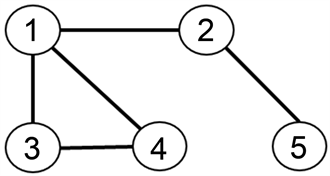Figure 1. Communication topology

$L=\left[\begin{array}{ccccc}3& -1& -1& -1& 0\\ -1& 2& 0& 0& -1\\ -1& 0& 2& -1& 0\\ -1& 0& -1& 2& 0\\ 0& -1& 0& 0& 1\end{array}\right],$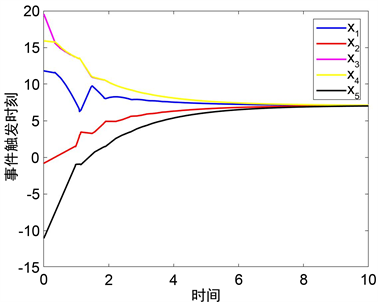Figure 2. States of the agents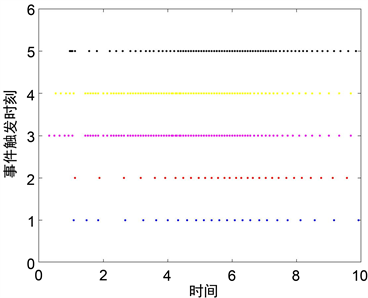Figure 3. Event-triggering time instants under (4)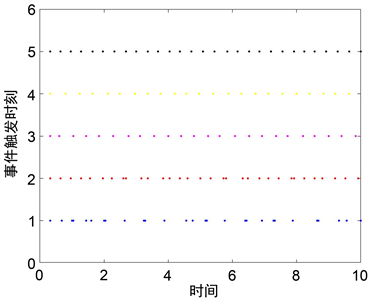Figure 4. Event-triggering time instants under (13)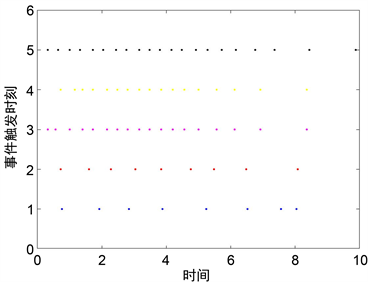Figure 5. Event-triggering time instants under (14)

5. 结论

 许文盈, 曹进德. 基于事件触发机制的多智能体系统协调控制研究综述[J]. 南京信息工程大学学报, 2018, 10(4): 395-400.

 徐鹏, 谢广明, 文家燕, 高远. 事件触发的强化学习多智能体编队控制[J]. 智能系统学报, 2019, 14(1): 93-98.

 Chen, J., Cao, X. and Cheng, P. (2010) Distributed Collaborative Control for Industrial Automation with Wireless Sensor and Actuator Networks. IEEE Transactions on Industrial Electronics, 57, 4219-4230.
https://doi.org/10.1109/TIE.2010.2043038

 Wang, X. and Yadav, V. (2007) Cooperative UAV Formation Flying with Obstacle Avoidance. IEEE Transactions on Control Systems Technology, 15, 672-679.
https://doi.org/10.1109/TCST.2007.899191

 Lin, W. (2014) Distributed UAV Formation Control Using Dif-ferential Game Approach. Aerospace Science and Technology, 35, 54-62.
https://doi.org/10.1016/j.ast.2014.02.004

 Savkin, A.V., Wang, C. and Baranzadeh, A. (2016) Distributed Formation Building Algorithms for Groups of Wheeled Mobile Robots. Robotics and Autonomous Systems, 75, 464-474.
https://doi.org/10.1016/j.robot.2015.08.006

 Dimos, V.D. and Karl, H.J. (2009) Event-Triggered Control for Multi-Agent Systems. 48th IEEE Conference on Decision and Control and 28th Chinese Control Conference, Shanghai, December 2009, 7131-7136.

 Georg, S.S., Dimos, V.D. and Karl, H.J. (2013) Event-Based Broadcasting for Multi-Agent Average Consensus. Automatica, 49, 245-252.
https://doi.org/10.1016/j.automatica.2012.08.042

 Fan, Y., Feng, G., Wang, Y. and Song, C. (2013) Distrib-uted Event-Triggered Control of Multi-Agent Systems with Combinational Measurements. Automatica, 49, 671-675.
https://doi.org/10.1016/j.automatica.2012.11.010

 Nowzari, C. and Cortes, J. (2014) Zeno-Free, Distributed Event-Triggered Communication and Control for Multi-Agent Average Consensus. American Control Conference (ACC), Portland, 4-6 June 2014, 2148-2153.
https://doi.org/10.1109/ACC.2014.6859495

 王航飞, 禹梅, 谢广明, 石红. 基于事件触发的环形编队多智能体系统[J]. 系统科学与数学, 2014, 34(7): 815-827.

 张志强, 王龙. 多智能体系统的事件触发控制[J]. 控制理论与应用, 2018, 35(8): 1051-1065.

 Meng, X., Xie, L., Soh, Y., Nowzari, C. and Pappas, G. (2015) Periodic Event-Triggered Average Consensus over Directed Graphs. Proceedings of the IEEE 54th Annual Conference on Decision and Control, Osaka, 15-18 December 2015, 4151-4156.
https://doi.org/10.1109/CDC.2015.7402866

 Meng, X. and Chen, T. (2013) Event Based Agreement Protocols for Multi-Agent Networks. Automatica, 49, 2125- 2132.
https://doi.org/10.1016/j.automatica.2013.03.002

 Fan, Y., Yang, Y. and Zhang, Y. (2016) Sampling-Based Event-Triggered Consensus for Multi-Agent Systems. Neurocomputing, 191, 141-147.
https://doi.org/10.1016/j.neucom.2015.12.102

 Wang, A. (2016) Event-Based Consensus Control for Single-Integrator Networks with Communication Time-Delays. Neurocomputing, 173, 1715-1719.
https://doi.org/10.1016/j.neucom.2015.09.044

 Liu, K. and Ji, Z. (2017) Consensus of Multi-Agent Systems with Time-Delay Based on Periodic Sample and Event Hybrid Control. Neurocomputing, 270, 11-17.
https://doi.org/10.1016/j.neucom.2016.12.106

 谢媛艳, 王毅, 马忠军. 领导-跟随多智能体系统的滞后一致性[J]. 物理学报, 2014, 63(4): 17-21.

 Wang, A. (2016) Event-Triggered Consensus Control for Lead-er-Following Multi-Agent Systems with Time-Varying Delays. Journal of the Franklin Institute, 353, 4754-4771.
https://doi.org/10.1016/j.jfranklin.2016.08.027

 Olfati-Saber, R. and Murray, R.M. (2004) Consensus Prob-lems in Networks of Agents with Switching Topology and Time-Delays. IEEE Transactions Automatic Control, 49, l520-l533.
https://doi.org/10.1109/TAC.2004.834113

Top